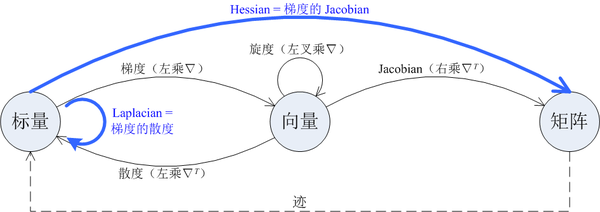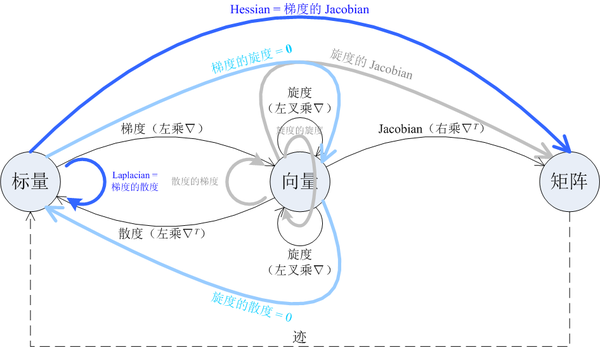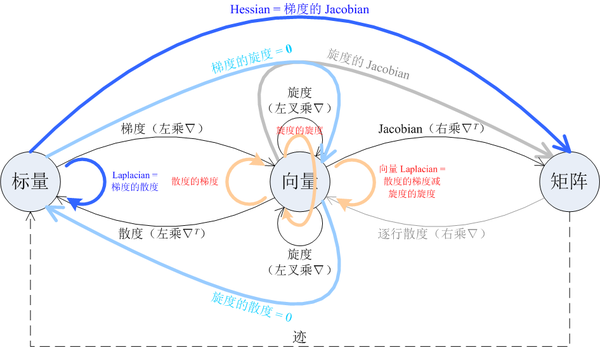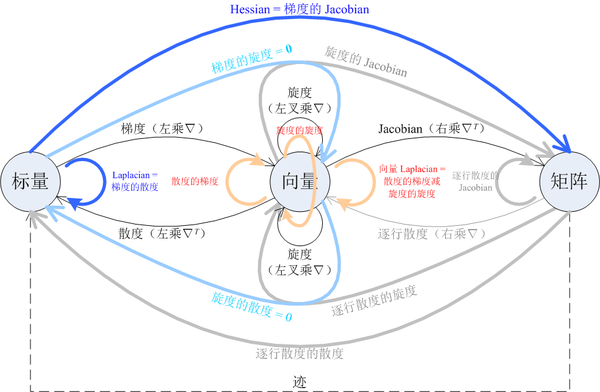# 化繁为简，一张图梳理梯度、散度、旋度、Jacobian、Hessian、Laplacian

## 一、入门图中的细实线箭头表示了四种一阶微分运算，包括梯度、散度、旋度和 Jacobian。每条箭头的起点表示了相应运算的自变量的类型，终点表示了相应运算的因变量的类型，例如梯度运算是作用在标量上的，结果是向量。图中的「向量」默认为列向量。

这四种一阶微分运算可以统一用算符 $\nabla$ （读作 nabla）表示。Nabla 算符是一个形式向量 $\nabla = \left[\frac{\partial}{\partial x} \quad \frac{\partial}{\partial y} \quad \frac{\partial}{\partial z} \right]^T$ ，它可以如下地作用于标量 $f$ 或向量 $\vec{v}$ 上：

• 直接与标量 $f$ 相乘，得到 $f$ 的梯度 $\nabla f$ 。
• 与向量 $\vec{v}$ 点乘，得到 $\vec{v}$ 的散度 $\nabla \cdot \vec{v}$ 。本文把点乘用矩阵乘法的形式写作 $\nabla^T \vec{v}$ 。
• 与向量 $\vec{v}$ 叉乘，得到 $\vec{v}$ 的旋度 $\nabla \times \vec{v}$ 。
• 若允许偏导算符写在变量的右边，则 $\vec{v}\nabla^T$ 就可以表示 $\vec{v}$ 的 Jacobian。

图中的粗实线箭头表示了两种二阶微分运算，它们可以由两个一阶微分运算组合而成，即：

• 梯度的散度就是 Laplacian；
• 梯度的 Jacobian 就是 Hessian。

图中的虚线箭头表示了一种不涉及微分的运算（迹）。在微分运算之后接上「迹」运算，可能得到另一种微分运算，如：

• Jacobian 的迹就是散度；
• Hessian 的迹就是 Laplacian。

## 二、入迷图中的四种一阶微分运算两两搭配，一共可以得到 7 种二阶微分运算。第一节的图中画出了两种，本节的图中画出了另外五种（浅蓝色与灰色）。这五种二阶微分运算并没有特别的名字，但其中有两种是恒等于 0 的：

• 梯度的旋度恒为零向量；
• 旋度的散度恒为 0。## 三、入魔Laplacian 是一个作用于标量的二阶微分运算，其结果也是标量。但我们也可以把它作用于一个向量的每一个元素，得到一个向量；这种运算称为向量 Laplacian。

Laplacian 运算作用于标量 $f$ 上的结果可以用 nabla 算符写成 $\nabla^T \nabla f$ 。这种写法无法直接推广到向量 Laplacian，因为 $\nabla^T \nabla \vec{v}$ 里 $\nabla$ 无法直接跟 $\vec{v}$ 做矩阵乘法。但如果允许偏导算符写在变量右边，那就可以把向量 Laplacian 表示成 $\vec{v} \nabla^T \nabla$ 。这是 Jacobian 运算与「矩阵右乘 $\nabla$ 」运算的复合；后者的效果是对矩阵的每一行求散度。图中恰好有一个为「逐行散度」运算准备的空位，我们把它补充到图中。

向量 Laplacian 的结果，恰好等于「散度的梯度」与「旋度的旋度」之差。为了体现出这种关系，我把「从向量到向量」的三种二阶微分运算改用橙红色箭头表示。

## 四、入土既然引入了「逐行散度」这个一阶微分运算，那就索性把它能组合出来的二阶微分运算也全都放到图里去吧！这样就得到了一个完美对称的图，它包含了 11 种二阶微分运算，其中：

• 有两种比较常见：Laplacian 和 Hessian；
• 有两种恒等于零：「梯度的旋度」和「旋度的散度」；
• 有三种满足减法关系：向量 Laplacian = 散度的梯度 - 旋度的旋度；
• 剩下的四种没有专门的名字，也很罕见。

其中任何一种微分运算后面接上「迹」，都可以得到另一种同阶微分运算：

• Jacobian 的迹就是散度；
• Hessian 的迹就是 Laplacian；
• 旋度的 Jacobian 的迹就是旋度的散度，恒等于 0；
• 矩阵逐行散度的 Jacobian 的迹，就是它的逐行散度的散度。

如果有读者知道图中几种没有名字的运算叫什么名字、有什么用途，或者在图中内容之外还有什么值得包括进来的微分运算，欢迎补充。